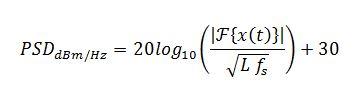# Power Spectral Density in MATLAB

Posted by in on 2-26-13

All real systems contain noise from various sources, be it from thermal noise, intentional or unintentional interference, cross-talk, etc. It’s important that we understand how to specify and model noise in our designs.

A noise concept that seems to come up often as a question to me is how to accurately evaluate the power spectral density in MATLAB, where the thermal noise floor specification given in dBm/Hz.

1. Thermal Noise Concepts
2. Mathematical Models
3. MATLAB Code for Power Spectral Density
4. M-Code Discussion

## Thermal Noise Concepts

We are used to thinking about noise in terms of a single power. What’s the carrier power of a modulated signal? How many Watts can the amplifier produce? What’s the interferer power inside the bandwidth of interest? At first exposure, a ratio like dBm/Hz is not as intuitive as a direct quantity.

Noise power is often given in terms of the ratio dBm/Hz — decibels with respect to mWatt per Hertz. Noise power is specified in this way so that emphasis is given to the noise conditions, and not the design. This is because the total noise power in a receiver is directly proportional to the bandwidth of the receiver. The wider your filters at the receiver input, the higher your thermal noise floor in the system. This can be seen in the following equation, giving the total noise power in a system:where k is the Boltzmann constant, T is the noise temperature of the system (room temperature is about 300 K), and B is the bandwidth of the system. You can see from the equation that increasing the bandwidth increases the total noise power.

Since thermal noise is often constant (or close to constant), and the bandwidth of a system can be designed to be nearly anything, specifications are typically given using the 10log10(kT) part of the equation (or assume the bandwidth is 1 Hz). That gives the noise specification in dBm/Hz! Adding 10log10(B) simply gives the total noise power in the system over the limited bandwidth, B.

## Mathematical Models for Power Spectral Density

There are a few different algorithms for estimating the power spectral density of a signal including a periodogram, Welch’s method, Yule-Walker Autoregressive Method, Burg Method, etc.

I used the word “estimate” intentionally because none of the PSD methods are perfect. Although the periodogram is based on methods that will be familiar to the average engineer, it doesn’t work as well for low signal-to-noise ratios (SNR) and suffers from higher variance.

For the sake of simplicity this article will stick to the periodogram, which is simple to implement in MATLAB. As long as you have a large number of time samples of the signal, and the SNR is sufficiently high, this method works fairly well.

The periodogram method of power spectral density estimation uses the fast Fourier transform (FFT) and is given by:where L is the number of samples of the signal x(t), and fs is the sampling frequency. We use 20log10 here instead of 10log10 because it is assumed that x(t) is a voltage, and we add 30 to the result because we want the result in dBm, not dBW.

## Power Spectral Density Plot in MATLAB

[cc lang=”matlab” noborder=”true” tab_size=”4″ lines=”-1″ width=”600″ escaped=”true”]
clear all

%% Define Parameters

% sampling frequency (Hz)
fs=100e6;

% length of time-domain signal
L=30e3;

% desired power specral density (dBm/Hz)
Pd=-100;

% number of FFT points
nfft=2^nextpow2(L);

% frequency plotting vector
f=fs/2*[-1:2/nfft:1-2/nfft];

% create
s=wgn(L,1,Pd+10*log10(fs),1,[],’dBm’,’complex’);

%% Analysis

% analyze spectrum
N=nfft/2+1:nfft;
S=fftshift(fft(s,nfft));
S=abs(S)/sqrt(L*fs);

% time-average for spectrum
Navg=4e2;
b(1:Navg)=1/Navg;
Sa=filtfilt(b,1,S);

% convert to dBm/Hz
S=20*log10(S)+30;
Sa=20*log10(Sa)+30;

%% Plot
figure(1)
clf
plot(f(N)/1e6,S(N))
hold on
plot(f(N)/1e6,Sa(N),’r’)
xlabel(‘Frequency (MHz)’)
ylabel(‘Power Density (dBm/Hz)’)
title([‘Power Spectral Density’])
legend(‘Noise Spectrum’,’Time-Averaged Spectrum’)
axis([10e-4 fs/2/1e6 -120 -60])
grid on
hold off
[/cc]## M-Code Discussion

Feel free to play around with different sampling frequencies and noise power densities to see how they change the plot. You can even copy the original noise signal, filter it, and add it back to the original to create “colored” noise that is not constant across the spectrum.

The wgn function is used in this m-code to create a random Gaussian noise signal of known power. The total power of the noise signal is specified using the first equation in this article.

The averaging shown in red on the plot is for illustration purposes, and to make it more clear where the noise floor is located. The averaging is done starting on line 31 using the filtfilt method. Averaging across the spectrum isn’t the most accurate method, but it’s pretty close, and good enough for the purpose of illustration.Did you enjoy this article?